# Kinetic energyThe cars of a roller coaster reach their maximum kinetic energy when at the bottom of their path. When they start rising, the kinetic energy begins to be converted to gravitational potential energy. The sum of kinetic and potential energy in the system remains constant, assuming negligible losses to friction.

The kinetic energy of an object is the extra energy it possesses due to its motion. It is defined as the work needed to accelerate a body of a given mass from rest to its current velocity. Having gained this energy during its acceleration, the body maintains this kinetic energy unless its speed changes. To return the body to a state of rest from that velocity, negative work of the same magnitude would be required.

Kinetic energy for single objects is completely frame-dependent (relative). For example, a bullet racing by a non-moving observer has kinetic energy in the reference frame of this observer, but the same bullet has zero kinetic energy in a reference frame that moves with the bullet. The kinetic energy of systems of objects, however, may sometimes not be completely removable by simple choice of reference frame. When this is the case, a residual minimum kinetic energy remains in the system as seen by all observers, and this kinetic energy (if present) contributes to the system's invariant mass, which is seen as the same value in all reference frames and by all observers.

The kinetic energy of flowing water or the wind can be used to move turbines, which in turn can be used to generate electricity.

## Etymology and history

The adjective "kinetic" has its roots in the Greek word κίνηση (kinesis) meaning "motion." The same root is used in the word cinema, referring to motion pictures.

The principle in classical mechanics that E ∝ mv² was first theorized by Gottfried Leibniz and Johann Bernoulli, who described kinetic energy as the "living force," or vis viva. Willem 's Gravesande of the Netherlands provided experimental evidence of this relationship. By dropping weights from different heights into a block of clay, 's Gravesande determined that their penetration depth was proportional to the square of their impact speed. Émilie du Châtelet recognized the implications of the experiment and published an explanation.

The terms "kinetic energy" and "work" with their present scientific meanings date back to the mid-nineteenth century. Early understandings of these ideas can be attributed to Gaspard-Gustave Coriolis, who in 1829 published a paper titled Du Calcul de l'Effet des Machines, outlining the mathematics of kinetic energy. William Thomson, later Lord Kelvin, is given credit for coining the term "kinetic energy," around 1849-1851.

## Introduction

There are various forms of energy, including chemical energy, heat, electromagnetic radiation, nuclear energy, and rest energy. They can be categorized in two main classes: potential energy and kinetic energy.

Kinetic energy can be best understood by examples that demonstrate how it is transformed to and from other forms of energy. For example, a cyclist will use chemical energy that was provided by food to accelerate a bicycle to a chosen speed. This speed can be maintained without further work, except to overcome air-resistance and friction. The energy has been converted into kinetic energy—the energy of motion—but the process is not completely efficient and heat is also produced within the cyclist.

The kinetic energy in the moving cyclist and the bicycle can be converted to other forms. For example, the cyclist could encounter a hill just high enough to coast up, so that the bicycle comes to a complete halt at the top. The kinetic energy has now largely been converted to gravitational potential energy that can be released by freewheeling down the other side of the hill. (Since the bicycle lost some of its energy to friction, it will never regain all of its speed without further pedaling. Note that the energy is not destroyed; it has only been converted to another form by friction.)

Alternatively, the cyclist could connect a dynamo to one of the wheels and also generate some electrical energy on the descent. The bicycle would be traveling more slowly at the bottom of the hill because some of the energy has been diverted into making electrical power. Another possibility would be for the cyclist to apply the brakes, in which case the kinetic energy would be dissipated through friction as heat energy.

Like any physical quantity that is a function of velocity, the kinetic energy of an object depends on the relationship between the object and the observer's frame of reference. Thus the kinetic energy of an object is not invariant.

### Examples

Spacecraft use chemical energy to take off and gain considerable kinetic energy to reach orbital velocity. This kinetic energy gained during launch will remain constant while in orbit because there is almost no friction. However, it becomes apparent at re-entry when the kinetic energy is converted to heat.

Kinetic energy can be passed from one object to another. In the game of billiards, the player gives kinetic energy to the cue ball by striking it with the cue stick. If the cue ball collides with another ball, it will slow down dramatically and the ball it collided with will accelerate to a speed as the kinetic energy is passed on to it. Collisions in billiards are effectively elastic collisions, where kinetic energy is preserved.

Flywheels are being developed as a method of energy storage (see Flywheel energy storage). This illustrates that kinetic energy can also be rotational.

### Calculations

There are several different equations that may be used to calculate the kinetic energy of an object. In many cases they give almost the same answer to well within measurable accuracy. Where they differ, the choice of which to use is determined by the velocity of the body or its size. Thus, if the object is moving at a velocity much smaller than the speed of light, the Newtonian (classical) mechanics will be sufficiently accurate; but if the speed is comparable to the speed of light, relativity starts to make significant differences to the result and should be used. If the size of the object is sub-atomic, the quantum mechanical equation is most appropriate.

## Newtonian kinetic energy

### Kinetic energy of rigid bodies

In classical mechanics, the kinetic energy of a "point object" (a body so small that its size can be ignored), or a non-rotating rigid body, is given by the equation$E_k = \begin{matrix} \frac{1}{2} \end{matrix} mv^2$

where m is the mass and v is the speed of the body. In SI units (used for most modern scientific work), mass is measured in kilograms, speed in metres per second, and the resulting kinetic energy is in joules.

For example, one would calculate the kinetic energy of an 80 kg mass traveling at 18 meters per second (40 mph) as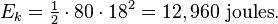$E_k = \begin{matrix} \frac{1}{2} \end{matrix} \cdot 80 \cdot 18^2 = 12,960 \ \mathrm{joules}.$

Note that the kinetic energy increases with the square of the speed. This means, for example, that an object traveling twice as fast will have four times as much kinetic energy. As a result of this, a car traveling twice as fast requires four times as much distance to stop (assuming a constant braking force. See mechanical work).

The kinetic energy of an object is related to its momentum by the equation: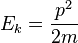$E_k = \frac{p^2}{2m}$

where p is momentum.

For the translational kinetic energy of a body with constant mass m, whose center of mass is moving in a straight line with speed v, as seen above is equal to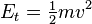$E_t = \begin{matrix} \frac{1}{2} \end{matrix} mv^2$

where:

m is mass of the body
v is speed of the center of mass of the body.

The kinetic energy of any entity is relative to the frame of reference in which it is measured. An isolated system (no energy can enter or leave) has a total energy which is unchanging over time as seen by any one observer. Thus, the chemical energy converted to kinetic energy by a rocket engine will be divided differently between the rocket ship and its exhaust stream depending upon the chosen frame of reference. But the total energy of the system (including kinetic energy, fuel chemical energy, heat energy, etc), will be conserved over time in a given reference frame, regardless of the choice of measurement frame. However, different observers will disagree on what the value of this conserved energy is.

In addition, although the energy of such systems is dependent on the measurement frame, the minimal total energy which is seen in any frame will be the total energy seen by observers in the center of momentum frame; this minimal energy corresponds to the invariant mass of the aggregate. The calculated value of this invariant mass compensates for changing energy in different frames, and is thus the same for all frames and observers.

#### Derivation

The work done accelerating a particle during the infinitesimal time interval dt is given by the dot product of force and displacement: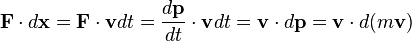$\mathbf{F} \cdot d \mathbf{x} = \mathbf{F} \cdot \mathbf{v} d t = \frac{d \mathbf{p}}{d t} \cdot \mathbf{v} d t = \mathbf{v} \cdot d \mathbf{p} = \mathbf{v} \cdot d (m \mathbf{v})$

Applying the product rule we see that: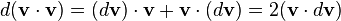$d(\mathbf{v} \cdot \mathbf{v}) = (d \mathbf{v}) \cdot \mathbf{v} + \mathbf{v} \cdot (d \mathbf{v}) = 2(\mathbf{v} \cdot d\mathbf{v})$

Therefore (assuming constant mass), the following can be seen: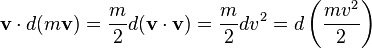$\mathbf{v} \cdot d (m \mathbf{v}) = \frac{m}{2} d (\mathbf{v} \cdot \mathbf{v}) = \frac{m}{2} d v^2 = d \left(\frac{m v^2}{2}\right)$

Since this is a total differential (that is, it only depends on the final state, not how the particle got there), we can integrate it and call the result kinetic energy: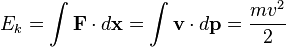$E_k = \int \mathbf{F} \cdot d \mathbf{x} = \int \mathbf{v} \cdot d \mathbf{p}= \frac{m v^2}{2}$

This equation states that the kinetic energy (Ek) is equal to the integral of the dot product of the velocity (v) of a body and the infinitesimal change of the body's momentum (p). It is assumed that the body starts with no kinetic energy when it is at rest (motionless).

### Rotating bodies

If a rigid body is rotating about any line through the center of mass then it has rotational kinetic energy ($E_r$) which is simply the sum of the kinetic energies of its moving parts, and is thus given by: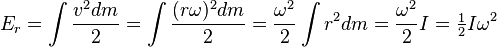$E_r = \int \frac{v^2 dm}{2} = \int \frac{(r \omega)^2 dm}{2} = \frac{\omega^2}{2} \int{r^2}dm = \frac{\omega^2}{2} I = \begin{matrix} \frac{1}{2} \end{matrix} I \omega^2$

where:

• ω is the body's angular velocity
• r is the distance of any mass dm from that line
•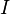$I$ is the body's moment of inertia, equal to$\int{r^2}dm$.

(In this equation the moment of inertia must be taken about an axis through the center of mass and the rotation measured by ω must be around that axis; more general equations exist for systems where the object is subject to wobble due to its eccentric shape).

### Kinetic energy of systems

A system of bodies may have internal kinetic energy due to macroscopic movements of the bodies in the system. For example, in the Solar System the planets and planetoids are orbiting the Sun. The kinetic energy of a system at any instant in time is the sum of the kinetic energies of the bodies it contains.

A body that is stationary and not rotating nevertheless has internal energy, which is partly kinetic energy, due to molecular translation, rotation, and vibration, electron translation and spin, and nuclear spin. When discussing movements of macroscopic bodies the kinetic energies referred to are usually those of the macroscopic movements only.

### Frame of reference

The total kinetic energy of a system depends on the inertial frame of reference: it is the sum of the total kinetic energy in a center of momentum frame and the kinetic energy the total mass would have if it were concentrated in the center of mass.

This may be simply shown: let V be the relative speed of the frame k from the center of mass frame i :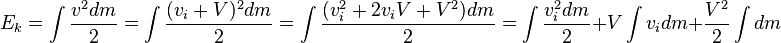$E_k = \int \frac{v^2 dm}{2} = \int \frac{(v_i + V)^2 dm}{2} = \int \frac{(v_i^2 + 2 v_i V + V^2) dm}{2} = \int \frac{v_i^2 dm}{2} + V \int v_i dm + \frac{V^2}{2} \int dm$

However, let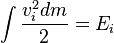$\int \frac{v_i^2 dm}{2} = E_i$ the kinetic energy in the center of mass frame,$\int v_i dm$ would be simply the total momentum which is by definition zero in the center of mass frame, and let the total mass: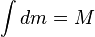$\int dm = M$. Substituting, we get:$E_k = E_i + \frac{M V^2}{2}$

Thus the kinetic energy of a system is lowest with respect to center of momentum reference frames, i.e., frames of reference in which the center of mass is stationary (either the center of mass frame or any other center of momentum frame). In any other frame of reference there is additional kinetic energy corresponding to the total mass moving at the speed of the center of mass. The kinetic energy of the system in the center of momentum frame contributes to the invariant mass of the system, and this total mass is a quantity which is both invariant (all observers see it to be the same) and is conserved (in an isolated system, it cannot change value, no matter what happens inside the system).

### Rotation in systems

It sometimes is convenient to split the total kinetic energy of a body into the sum of the body's center-of-mass translational kinetic energy and the energy of rotation around the center of mass rotational energy: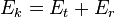$E_k = E_t + E_r \,$

where:

Ek is the total kinetic energy
Et is the translational kinetic energy
Er is the rotational energy or angular kinetic energy in the rest frame

Thus the kinetic energy of a tennis ball in flight is the kinetic energy due to its rotation, plus the kinetic energy due to its translation.

## Relativistic kinetic energy of rigid bodies

In special relativity, we must change the expression for linear momentum. Integrating by parts, we get: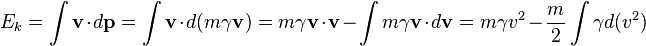$E_k = \int \mathbf{v} \cdot d \mathbf{p}= \int \mathbf{v} \cdot d (m \gamma \mathbf{v}) = m \gamma \mathbf{v} \cdot \mathbf{v} - \int m \gamma \mathbf{v} \cdot d \mathbf{v} = m \gamma v^2 - \frac{m}{2} \int \gamma d (v^2)$

Remembering that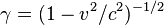$\gamma = (1 - v^2/c^2)^{-1/2}\!$, we get:$E_k = m \gamma v^2 - \frac{- m c^2}{2} \int \gamma d (1 - v^2/c^2) = m \gamma v^2 + m c^2 (1 - v^2/c^2)^{1/2} + C$

And thus: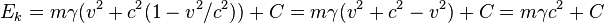$E_k = m \gamma (v^2 + c^2 (1 - v^2/c^2)) + C = m \gamma (v^2 + c^2 - v^2) + C = m \gamma c^2 + C\!$

The constant of integration is found by observing that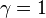$\gamma = 1\!$ and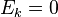$E_k = 0 \!$ when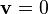$\mathbf{v }= 0$, so we get the usual formula: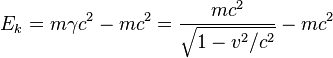$E_k = m \gamma c^2 - m c^2 = \frac{m c^2}{\sqrt{1 - v^2/c^2}} - m c^2$

If a body's speed is a significant fraction of the speed of light, it is necessary to use relativistic mechanics (the theory of relativity as expounded by Albert Einstein) to calculate its kinetic energy.

For a relativistic object the momentum p is equal to: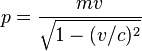$p = \frac{m v}{\sqrt{1 - (v/c)^2}}$,

where m is the rest mass, v is the object's speed, and c is the speed of light in vacuum.

Thus the work expended accelerating an object from rest to a relativistic speed is: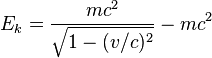$E_k = \frac{m c^2}{\sqrt{1 - (v/c)^2}} - m c^2$.

The equation shows that the energy of an object approaches infinity as the velocity v approaches the speed of light c, thus it is impossible to accelerate an object across this boundary.

The mathematical by-product of this calculation is the mass-energy equivalence formula—the body at rest must have energy content equal to: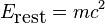$E_\mbox{rest} = m c^2 \!$

At a low speed (v<<c), the relativistic kinetic energy may be approximated well by the classical kinetic energy. This is done by binomial approximation. Indeed, taking Taylor expansion for square root and keeping first two terms it calculates as: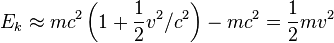$E_k \approx m c^2 \left(1 + \frac{1}{2} v^2/c^2\right) - m c^2 = \frac{1}{2} m v^2$,

So, the total energy E can be partitioned into the energy of the rest mass plus the traditional Newtonian kinetic energy at low speeds.

When objects move at a speed much slower than light (e.g. in everyday phenomena on Earth), the first two terms of the series predominate. The next term in the approximation is small for low speeds, and can be found by extending the expansion into a Taylor series by one more term:$E \approx m c^2 \left(1 + \frac{1}{2} v^2/c^2 + \frac{3}{8} v^4/c^4\right) = m c^2 + \frac{1}{2} m v^2 + \frac{3}{8} m v^4/c^2$.

For example, for a speed of 10 km/s the correction to the Newtonian kinetic energy is 0.07 J/kg (on a Newtonian kinetic energy of 50 MJ/kg) and for a speed of 100 km/s it is 710 J/kg (on a Newtonian kinetic energy of 5 GJ/kg), etc.

For higher speeds, the formula for the relativistic kinetic energy is derived by simply subtracting the rest mass energy from the total energy:$E_k = m \gamma c^2 - m c^2 = m c^2\left(\frac{1}{\sqrt{1 - (v/c)^2}} - 1\right)$.

The relation between kinetic energy and momentum is more complicated in this case, and is given by the equation: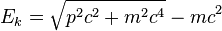$E_k = \sqrt{p^2 c^2 + m^2 c^4} - m c^2$.

This can also be expanded as a Taylor series, the first term of which is the simple expression from Newtonian mechanics.

What this suggests is that the formulas for energy and momentum are not special and axiomatic, but rather concepts which emerge from the equation of mass with energy and the principles of relativity.

## Quantum mechanical kinetic energy of rigid bodies

In the realm of quantum mechanics, the expectation value of the electron kinetic energy,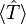$\langle\hat{T}\rangle$, for a system of electrons described by the wavefunction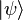$\vert\psi\rangle$ is a sum of 1-electron operator expectation values: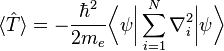$\langle\hat{T}\rangle = -\frac{\hbar^2}{2 m_e}\bigg\langle\psi \bigg\vert \sum_{i=1}^N \nabla^2_i \bigg\vert \psi \bigg\rangle$

where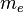$m_e$ is the mass of the electron and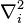$\nabla^2_i$ is the Laplacian operator acting upon the coordinates of the ith electron and the summation runs over all electrons. Notice that this is the quantized version of the non-relativistic expression for kinetic energy in terms of momentum: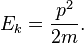$E_k = \frac{p^2}{2m}.$

The density functional formalism of quantum mechanics requires knowledge of the electron density only, i.e., it formally does not require knowledge of the wavefunction. Given an electron density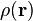$\rho(\mathbf{r})$, the exact N-electron kinetic energy functional is unknown; however, for the specific case of a 1-electron system, the kinetic energy can be written as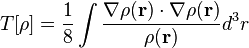$T[\rho] = \frac{1}{8} \int \frac{ \nabla \rho(\mathbf{r}) \cdot \nabla \rho(\mathbf{r}) }{ \rho(\mathbf{r}) } d^3r$

where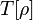$T[\rho]$ is known as the von Weizsäcker kinetic energy functional.